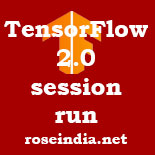# TensorFlow 2.0 session run

In TensorFlow 2.0 the session has been removed and there is no session run method in this version of TensorFlow.## TensorFlow 2.0 session run - removed

In TensorFlow 2.0 session has been removed and now the code is executed by TensorFlow 2.0 sequentially in the python code. This has been removed as we have eager execution is enabled by default.

For example if we have following code in TensorFlow 1.x which uses session:

``````
in_a = tf.placeholder(dtype=tf.float32, shape=(2))
in_b = tf.placeholder(dtype=tf.float32, shape=(2))

def forward(x):
with tf.variable_scope("matmul", reuse=tf.AUTO_REUSE):
W = tf.get_variable("W", initializer=tf.ones(shape=(2,2)),
regularizer=tf.contrib.layers.l2_regularizer(0.04))
b = tf.get_variable("b", initializer=tf.zeros(shape=(2)))
return W * x + b

out_a = forward(in_a)
out_b = forward(in_b)

reg_loss = tf.losses.get_regularization_loss(scope="matmul")

with tf.Session() as sess:
sess.run(tf.global_variables_initializer())
outs = sess.run([out_a, out_b, reg_loss],
feed_dict={in_a: [1, 0], in_b: [0, 1]})

``````

Above code is valid in TensorFlow 1.x but now we don't have tf.Session() method. Then the tf.Session().run() must be changed to the Python function. After changing the above code it will look like below for TensorFlow 2.0:

``````
import tensorflow as tf
W = tf.Variable(tf.ones(shape=(2,2)), name="W")
b = tf.Variable(tf.zeros(shape=(2)), name="b")

@tf.function
def forward(x):
return W * x + b

out_a = forward([1,0])
print(out_a)

tf.print(out_a)
``````

So, we have to change the tf.Session() to a Python function in TensorFlow 2.0. If you run the above program it will give following output:

``````
tf.Tensor(
[[1. 0.]
[1. 0.]], shape=(2, 2), dtype=float32)
[[1 0]
[1 0]]

``````

Here is the output of program when executed in the Google Colab environment:In TensorFlow 2.0 Session has been completely removed. If you try to use it in your program then it will give following error:

``````
AttributeError                            Traceback (most recent call last)
<ipython-input-3-f75057d1d95f> in <module>()
----> 1 sess = tf.Session()

AttributeError: module 'tensorflow' has no attribute 'Session'
``````

So, We have to convert the code inside Session().run() to a Python function in TensorFlow 2.0.

You can find more tutorials at: